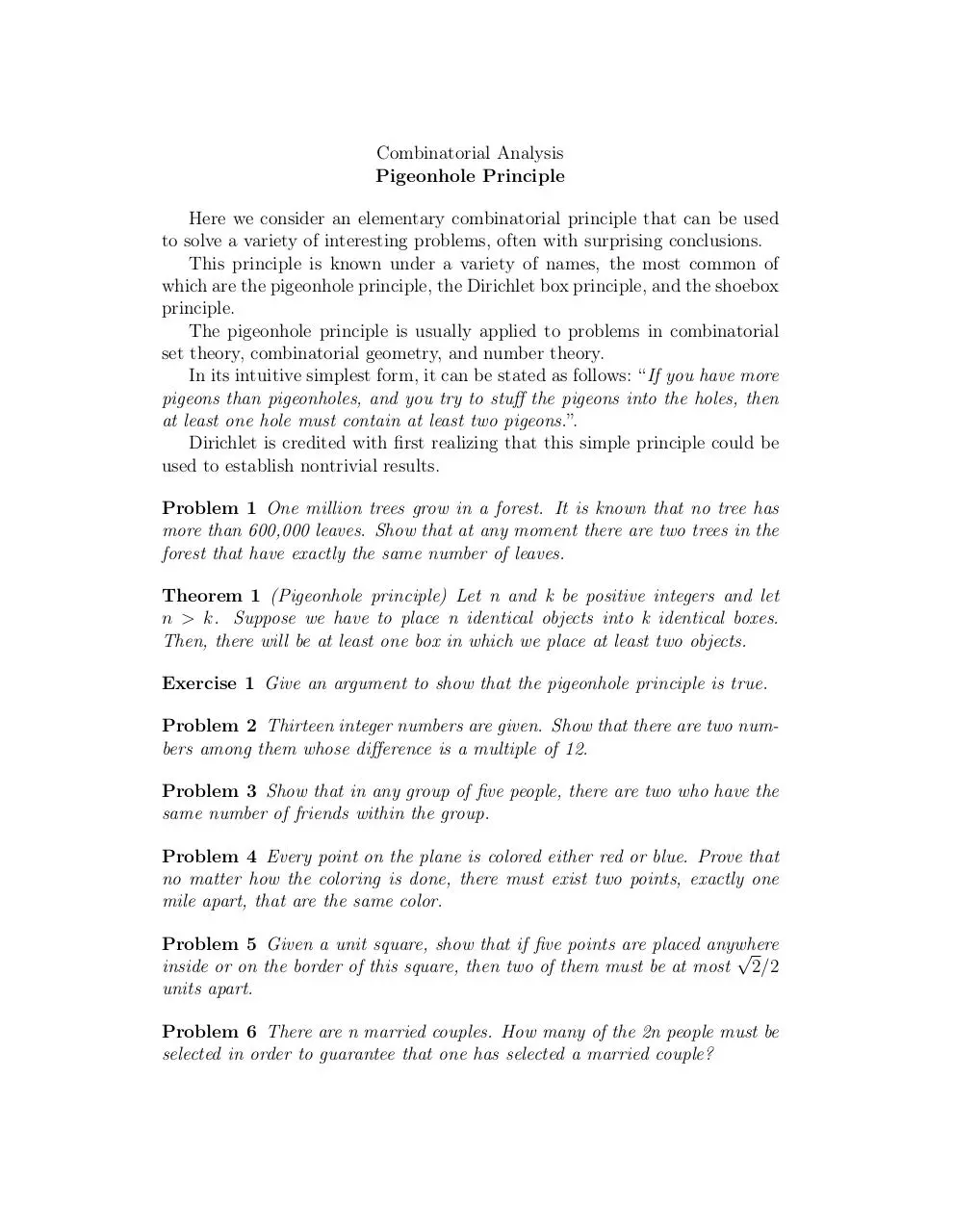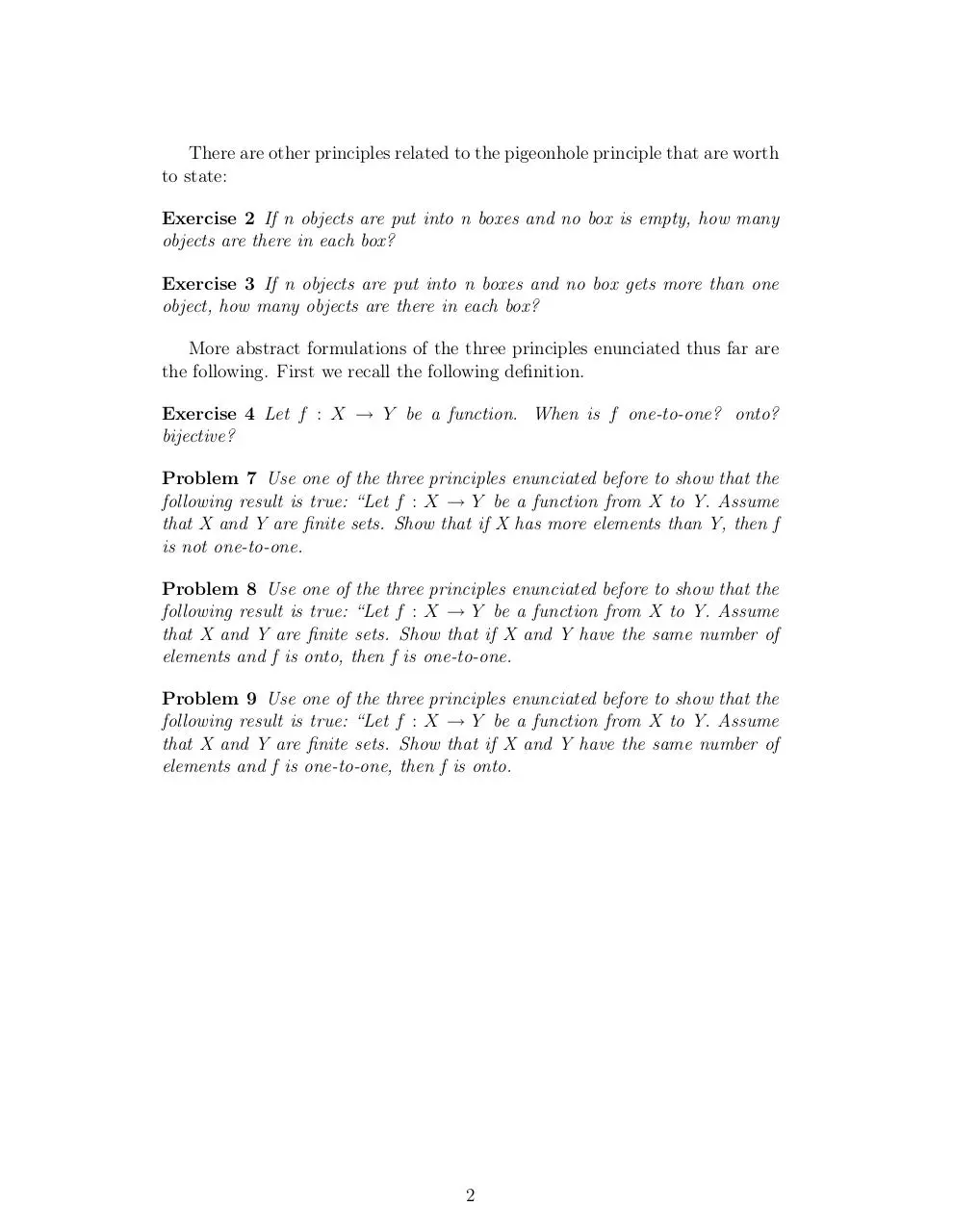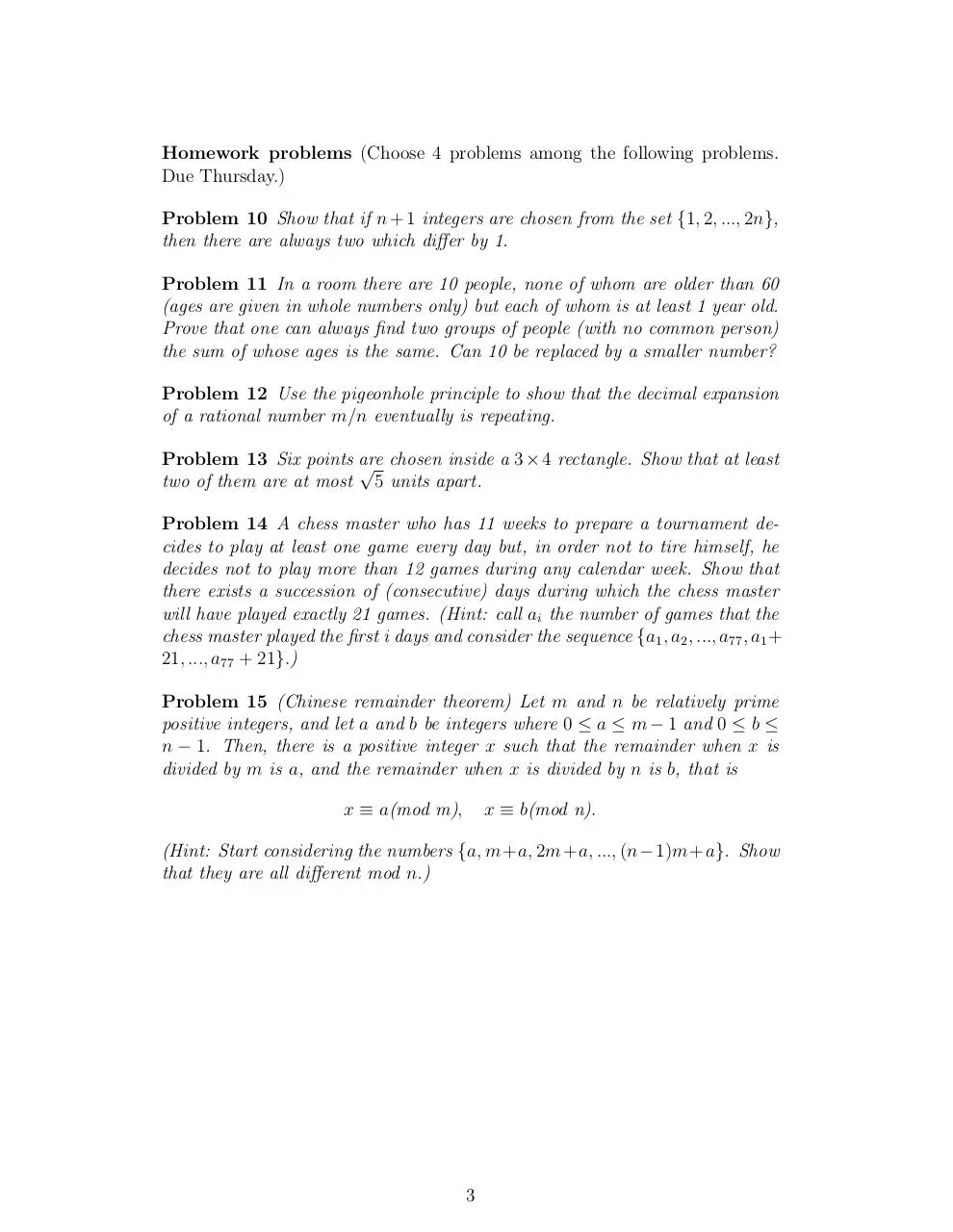# Day 2 Pigeonhole (PDF)

### File information

This PDF 1.4 document has been generated by TeX / pdfTeX-1.40.3, and has been sent on pdf-archive.com on 24/01/2014 at 06:06, from IP address 169.231.x.x. The current document download page has been viewed 1010 times.
File size: 94.42 KB (3 pages).
Privacy: public file### File preview

Combinatorial Analysis
Pigeonhole Principle
Here we consider an elementary combinatorial principle that can be used
to solve a variety of interesting problems, often with surprising conclusions.
This principle is known under a variety of names, the most common of
which are the pigeonhole principle, the Dirichlet box principle, and the shoebox
principle.
The pigeonhole principle is usually applied to problems in combinatorial
set theory, combinatorial geometry, and number theory.
In its intuitive simplest form, it can be stated as follows: “If you have more
pigeons than pigeonholes, and you try to stuff the pigeons into the holes, then
at least one hole must contain at least two pigeons.”.
Dirichlet is credited with first realizing that this simple principle could be
used to establish nontrivial results.
Problem 1 One million trees grow in a forest. It is known that no tree has
more than 600,000 leaves. Show that at any moment there are two trees in the
forest that have exactly the same number of leaves.
Theorem 1 (Pigeonhole principle) Let n and k be positive integers and let
n &gt; k. Suppose we have to place n identical objects into k identical boxes.
Then, there will be at least one box in which we place at least two objects.
Exercise 1 Give an argument to show that the pigeonhole principle is true.
Problem 2 Thirteen integer numbers are given. Show that there are two numbers among them whose difference is a multiple of 12.
Problem 3 Show that in any group of five people, there are two who have the
same number of friends within the group.
Problem 4 Every point on the plane is colored either red or blue. Prove that
no matter how the coloring is done, there must exist two points, exactly one
mile apart, that are the same color.
Problem 5 Given a unit square, show that if five points are placed anywhere

inside or on the border of this square, then two of them must be at most 2/2
units apart.
Problem 6 There are n married couples. How many of the 2n people must be
selected in order to guarantee that one has selected a married couple?

There are other principles related to the pigeonhole principle that are worth
to state:
Exercise 2 If n objects are put into n boxes and no box is empty, how many
objects are there in each box?
Exercise 3 If n objects are put into n boxes and no box gets more than one
object, how many objects are there in each box?
More abstract formulations of the three principles enunciated thus far are
the following. First we recall the following definition.
Exercise 4 Let f : X → Y be a function. When is f one-to-one? onto?
bijective?
Problem 7 Use one of the three principles enunciated before to show that the
following result is true: “Let f : X → Y be a function from X to Y. Assume
that X and Y are finite sets. Show that if X has more elements than Y, then f
is not one-to-one.
Problem 8 Use one of the three principles enunciated before to show that the
following result is true: “Let f : X → Y be a function from X to Y. Assume
that X and Y are finite sets. Show that if X and Y have the same number of
elements and f is onto, then f is one-to-one.
Problem 9 Use one of the three principles enunciated before to show that the
following result is true: “Let f : X → Y be a function from X to Y. Assume
that X and Y are finite sets. Show that if X and Y have the same number of
elements and f is one-to-one, then f is onto.

2

Homework problems (Choose 4 problems among the following problems.
Due Thursday.)
Problem 10 Show that if n + 1 integers are chosen from the set {1, 2, ..., 2n},
then there are always two which differ by 1.
Problem 11 In a room there are 10 people, none of whom are older than 60
(ages are given in whole numbers only) but each of whom is at least 1 year old.
Prove that one can always find two groups of people (with no common person)
the sum of whose ages is the same. Can 10 be replaced by a smaller number?
Problem 12 Use the pigeonhole principle to show that the decimal expansion
of a rational number m/n eventually is repeating.
Problem 13 Six points are
√ chosen inside a 3 × 4 rectangle. Show that at least
two of them are at most 5 units apart.
Problem 14 A chess master who has 11 weeks to prepare a tournament decides to play at least one game every day but, in order not to tire himself, he
decides not to play more than 12 games during any calendar week. Show that
there exists a succession of (consecutive) days during which the chess master
will have played exactly 21 games. (Hint: call ai the number of games that the
chess master played the first i days and consider the sequence {a1 , a2 , ..., a77 , a1 +
21, ..., a77 + 21}.)
Problem 15 (Chinese remainder theorem) Let m and n be relatively prime
positive integers, and let a and b be integers where 0 ≤ a ≤ m − 1 and 0 ≤ b ≤
n − 1. Then, there is a positive integer x such that the remainder when x is
divided by m is a, and the remainder when x is divided by n is b, that is
x ≡ a(mod m),

x ≡ b(mod n).

(Hint: Start considering the numbers {a, m+a, 2m+a, ..., (n−1)m+a}. Show
that they are all different mod n.)

3

Day 2- Pigeonhole.pdf (PDF, 94.42 KB)

#### HTML Code

Copy the following HTML code to share your document on a Website or Blog